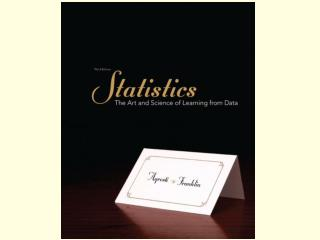DownloadDownload PresentationChapter 2 Exploring Data with Graphs and Numerical Summaries

# Chapter 2 Exploring Data with Graphs and Numerical Summaries

Télécharger la présentation## Chapter 2 Exploring Data with Graphs and Numerical Summaries

- - - - - - - - - - - - - - - - - - - - - - - - - - - E N D - - - - - - - - - - - - - - - - - - - - - - - - - - -
##### Presentation Transcript

1. Chapter 2Exploring Data with Graphs and Numerical Summaries Section 2.1 Different Types of Data

2. A variable is any characteristic observed in a study. Examples: Marital status, Height, Weight, IQ A variable can be classified as either Categorical (in Categories), or Quantitative (Numerical) Variable

3. A variable can be classified as categorical if each observation belongs to one of a set of categories: Examples: Gender (Male or Female) Religious Affiliation (Catholic, Jewish, …) Type of Residence (Apartment, Condo, …) Belief in Life After Death (Yes or No) Categorical Variable

4. A variable is called quantitative if observations on it take numerical values that represent different magnitudes of the variable. Examples: Age Number of Siblings Annual Income Quantitative Variable

5. For Quantitative variables: key features are the center and spread (variability) of the data. Example: What’s a typical annual amount of precipitation? Is there much variation from year to year? For Categorical variables: a key feature is the percentage of observations in each of the categories. Example: What percentage of students at a certain college are Democrats? Main Features of Quantitative and Categorical Variables

6. A quantitative variable is discrete if its possible values form a set of separate numbers, such as 0,1,2,3,…. Discrete variables have a finite number of possible values. Examples: Number of pets in a household Number of children in a family Number of foreign languages spoken by an individual Discrete Quantitative Variable

7. A quantitative variable is continuous if its possible values form an interval. Continuous variables have an infinite number of possible values. Examples: Height/Weight Age Blood pressure Continuous Quantitative Variable

8. Identify the variable type as either categorical or quantitative. Number of siblings in a family County of residence Distance (in miles) of commute to school Marital status Class Problem #1

9. Identify each of the following variables as continuous or discrete. Length of time to take a test Number of people waiting in line Number of speeding tickets received last year Your dog’s weight Class Problem #2

10. The proportion of the observations that fall in a certain category is the frequency (count) of observations in that category divided by the total number of observations. Frequency of that class Sum of all frequencies The percentage is the proportion multiplied by 100. Proportions and percentages are also called relative frequencies. Proportion & Percentage (Relative Frequencies)

11. There were 268 reported shark attacks in Florida between 2000 and 2010. Table 2.1 classifies 715 shark attacks reported from 2000 through 2010, so, for Florida, 268 is the frequency. 0.375 =268/715 is the proportion and relative frequency. 37.5 is the percentage 0.375 *100= 37.5%. Frequency, Proportion, & Percentage Example

12. A frequency table is a listing of possible values for a variable, together with the number of observations and/or relative frequencies for each value. Frequency Table Table 2.1 Frequency of Shark Attacks in Various Regions for 2000–2010

13. A stock broker has been following different stocks over the last month and has recorded whether a stock is up, the same, or down in value. The results were: What is the variable of interest? What type of variable is it? Add proportions to this frequency table. Class Problem #3